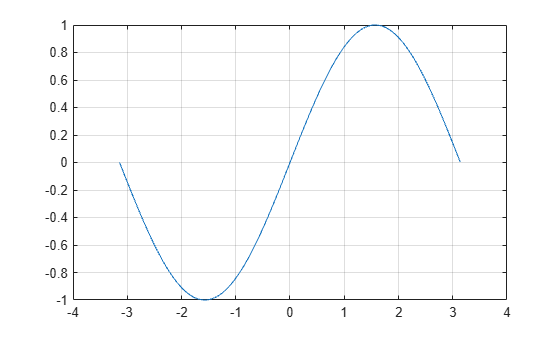# sin

## Syntax

``Y = sin(X)``

## Description

example

````Y = sin(X)` returns the sine of the elements of `X`. The `sin` function operates element-wise on arrays. The function accepts both real and complex inputs. For real values of `X`, `sin(X)` returns real values in the interval [-1, 1].For complex values of `X`, `sin(X)` returns complex values. ```

## Examples

collapse all

Plot the sine function over the domain $-\pi \le x\le \pi$.

```x = -pi:0.01:pi; plot(x,sin(x)), grid on```Calculate the sine of the complex angles in vector `x`.

```x = [-i pi+i*pi/2 -1+i*4]; y = sin(x)```
```y = 1×3 complex 0.0000 - 1.1752i 0.0000 - 2.3013i -22.9791 +14.7448i ```

## Input Arguments

collapse all

Input angle in radians, specified as a scalar, vector, matrix, multidimensional array, table, or timetable.

Data Types: `single` | `double` | `table` | `timetable`
Complex Number Support: Yes

## Output Arguments

collapse all

Sine of input angle, returned as a real-valued or complex-valued scalar, vector, matrix, multidimensional array, table, or timetable.

collapse all

### Sine Function

The sine of an angle, α, defined with reference to a right triangle isThe sine of a complex argument, α, is

`$\mathrm{sin}\left(\alpha \right)=\frac{{e}^{i\alpha }-{e}^{-i\alpha }}{2i}\text{\hspace{0.17em}}.$`

## Tips

• To compute `sin(X*pi)` accurately, without using `pi` as a floating-point approximation of π, you can use the `sinpi` function instead. For example, `sinpi(n)` is exactly zero for integers `n` and `sinpi(m/2)` is +1 or –1 for odd integers `m`.

## Version History

Introduced before R2006a

expand all